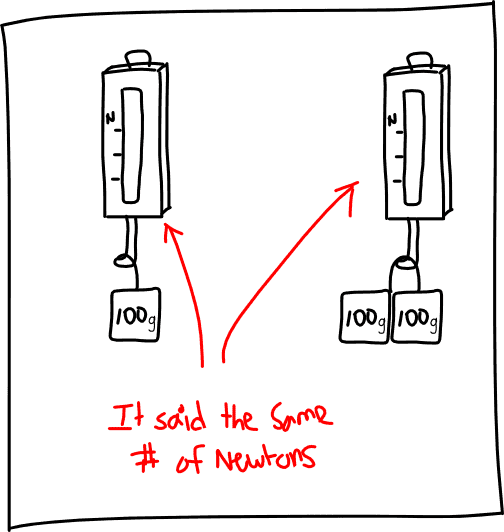# Tension and pulleys (not calculative questions)

## Homework Statement

1) What force does a spring scale measure? (I measured several different masses in different ways using pulleys and the spring scale always came up with the same number of Newtons, is this tension or what?)

2) Why, even when two different sized masses are hanged upon a pulley, the spring scale comes up with the same number of Newtons? What does this say about the tension in the spring?

3) If two masses are hanged upon a pulley and one of them is greater, will the smaller one accelerate? If so, in what direction? What about the bigger one? How do they compare in magnitude, and explain this.

## The Attempt at a Solution

1) I think it's the tension but I'm not sure.

2) I have no clue.

3) I believe the smaller one will accelerate upwards and the bigger one downwards but I don't know why and I don't know if the |acceleration| is equal.

Any help at all?

PhanthomJay
Homework Helper
Gold Member
Do you have a sketch or description? a spring scale measures force...if it is installed in the cord, it measures the cord tension....but i don't understand why the tension would be the same when you change the masses....please describe problem in detail.

So is it tension that it's measuring or the net force (including the force of gravity)?

Here's a diagram:PhanthomJay